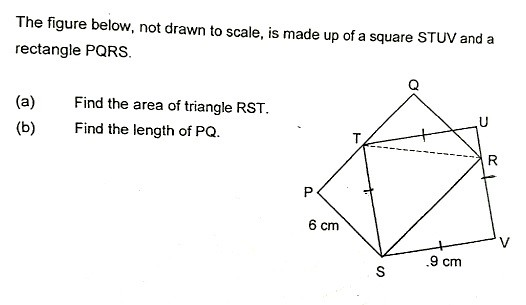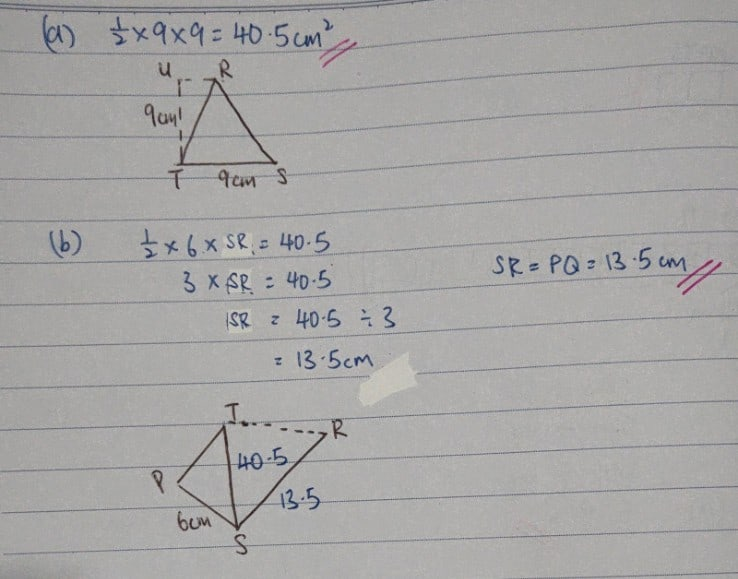QuestionThis is a 3 marks question.. Is the below solution correct??

Triangle RST is an equilateral triangle.

ST = 9cm. So all sides are length 9cm. Height = 6cm.

Area of triangle RST = ½ x 9 x 6 = 27cm2

Rectangle PQSU, length = 9cm, since SR = 9cm.

Ans: 27cm2, 9cm